• Java版本实现9宫格或者多宫格的两点距离最短的算法。各种大厂的面试题中的最基本算法知识，实现两点之间的最短距离实现方法。
• importjava.util.Scanner;//最短路径求解public classDistMin {static classGraphMatrix{static final int MaxNum=20;char[] Vertex=new char[MaxNum]; //保存顶点信息(序号或字母)int GType; //图的类型(0：...
packagecom.cn.datastruct;importjava.util.Scanner;//最短路径求解public classDistMin {static classGraphMatrix{static final int MaxNum=20;char[] Vertex=new char[MaxNum];  //保存顶点信息(序号或字母)int GType;    //图的类型(0：无向图，1：有向图)int VertexNum;        //顶点的数量int EdgeNum;        //边的数量int[][] EdgeWeight=new int[MaxNum][MaxNum];        //保存边的权int[] isTrav=new int[MaxNum];        //遍历标志}static final int MaxValue=65535;    //最大值(可设为一个最大整数)static int[] path=new int[GraphMatrix.MaxNum];    //两点经过的顶点集合的数组static int[] tmpvertex=new int[GraphMatrix.MaxNum];  //最短路径的起始点集合static Scanner input=newScanner(System.in);//创建邻接矩阵图static voidCreateGraph(GraphMatrix GM){inti,j,k;int weight;     //权char EstartV,EendV;        //边的起始顶点System.out.printf("输入图中各顶点信息\n");for(i=0;iSystem.out.printf("第%d个顶点：", i+1);GM.Vertex[i]=(input.next().toCharArray());  //保存到各顶点的数组元素中}System.out.printf("输入构成各边的顶点及权值：\n");for(k=0;kSystem.out.printf("第%d条边：", k+1);EstartV=input.next().charAt(0);EendV=input.next().charAt(0);weight=input.nextInt();for(i=0;EstartV!=GM.Vertex[i];i++);      //在已有顶点中查找始点for(j=0;EendV!=GM.Vertex[j];j++);        //在已有的顶点中查找终点GM.EdgeWeight[i][j]=weight;          //对应位置保存权值，表示有一条边if(GM.GType==0){        //若是无向图GM.EdgeWeight[j][i]=weight;        //在对角位置保存权值}}}//清空矩阵static voidClearGraph(GraphMatrix GM) {inti, j;for (i = 0; i < GM.VertexNum; i++) {for (j = 0; j < GM.VertexNum; j++) {GM.EdgeWeight[i][j]= MaxValue; //设置矩阵中各元素的值为MaxValue}}}//输出邻接矩阵static voidOutGraph(GraphMatrix GM) {inti, j;for (j = 0; j < GM.VertexNum; j++) {System.out.printf("\t%c", GM.Vertex[j]); //在第一行输出顶点信息}System.out.println();for (i = 0; i < GM.VertexNum; i++) {System.out.printf("%c", GM.Vertex[i]);for (j = 0; j < GM.VertexNum; j++) {if (GM.EdgeWeight[i][j] == MaxValue) { //若权值为最大值System.out.printf("\tZ"); //以Z表示无穷大} else{System.out.printf("\t%d", GM.EdgeWeight[i][j]); //输出边的权值}}System.out.println();}}//最短路径算法static void distMin(GraphMatrix GM,int vend){    //vend为结束点int[] weight=new int[GraphMatrix.MaxNum];        //某终止点到各顶点的最短路径长度inti,j,k,min;vend--;for(i=0;iweight[i]=GM.EdgeWeight[vend][i];}for(i=0;iif(weight[i]0){    //有效权值path[i]=vend;}}for(i=0;itmpvertex[i]=0;            //初始化顶点集合为空}tmpvertex[vend]=1;        //选入顶点vendweight[vend]=0;for(i=0;imin=MaxValue;k=vend;for(j=0;jmin=weight[j];k=j;}}tmpvertex[k]=1;            //将顶点k选入for(j=0;jif(tmpvertex[j]==0&&weight[k]+GM.EdgeWeight[k][j]weight[j]=weight[k]+GM.EdgeWeight[k][j];path[j]=k;}}}}public static voidmain(String[] args) {GraphMatrix GM=new GraphMatrix();   //定义保存邻接表结构的图String go;intvend;inti,k;System.out.println("求解最短路径问题！");do{System.out.print("请先输入生成图的类型：");GM.GType=input.nextInt();   //图的种类System.out.print("请输入图的顶点数量：");GM.VertexNum=input.nextInt();         //输入图中顶点数System.out.print("请输入图的边的数量：");GM.EdgeNum=input.nextInt();        //输入图中边数ClearGraph(GM);            //清空图CreateGraph(GM);        //生成邻接表结构的图System.out.print("\n请输入结束点：");vend=input.nextInt();distMin(GM,vend);vend--;System.out.printf("\n个顶点到达顶点%c的最短路径分别为(起始点-结束点)：\n",GM.Vertex[vend]);for(i=0;iif(tmpvertex[i]==1){k=i;while(k!=vend){System.out.printf("顶点%c-", GM.Vertex[k]);k=path[k];}System.out.printf("顶点%c\n", GM.Vertex[k]);}else{System.out.printf("%c-%c:无路径\n", GM.Vertex[i],GM.Vertex[vend]);}}System.out.println("\n继续玩吗(y/n)?");go=input.next();}while(go.equalsIgnoreCase("y"));System.out.println("游戏结束！");}}
展开全文• 这其实课看成球面上任意两点之间的最短距离。过球面上的任意两点以及球心可以做一个截平面，与球面的截痕为一个圆，这个圆的大小不随两点不同而变化，半径都是球半径。这个圆是任意平面与球面相截得到的所有不同的圆...
为何地图上的航线是曲线如果我们观察地图上的航线，就会发现航线是弯曲的。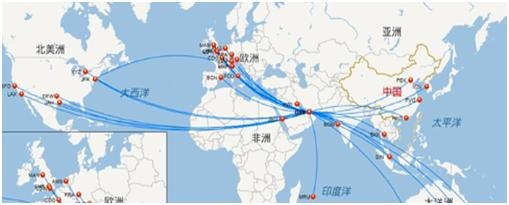基本上可以认为地球是个球体，如果飞机在两个城市之间飞行，最好的飞行线路是取这两个城市之间的最短距离。这其实课看成球面上任意两点之间的最短距离。过球面上的任意两点以及球心可以做一个截平面，与球面的截痕为一个圆，这个圆的大小不随两点不同而变化，半径都是球半径。这个圆是任意平面与球面相截得到的所有不同的圆中，半径最大的，因此叫做大圆。而只要你沿着球表面做线连接任意两个点，曲线长度最短的一定是这个大圆的劣弧长度。航线按两个城市之间的大圆弧航行才最经济。地图是球面向平面做投影做出来的，所以我们看到的航线就是曲线了。定理：球面上任意两点间的距离以大圆最短初等几何的观察如图AB是连接A,B两点的大圆弧，C是AB弧上的任意一点，过C做以A,B为极点的圆,设AF,GF，GB为一条球面曲线，且BG是大圆弧，AF也是大圆弧则CB=BG,AC=AF,但AF+FG+GB>AF+GB=AC+CB=AB.如果B,E,D,A是另外一条球面上的曲线，过B,D,A的球面三角形中AD+BD>AB,过E,B,A的球面三角形中亦有BE+AE>AB。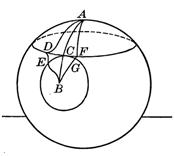微积分证明 下面我们利用球面坐标系与微积分给出一个精确的证明。令A,B是半径为R的球面上的任意两点，C为球心，大圆弧长可以表达为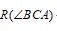以C为中心建立直角坐标系，让A在z轴上，则球面上任意一点P的坐标可以写成：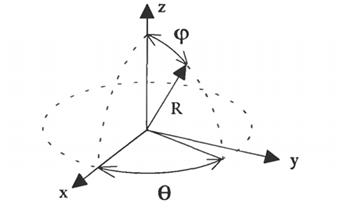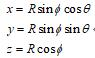空间中任意曲线的长度可以定义为：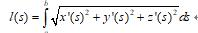其中s是参数，对球面曲线就有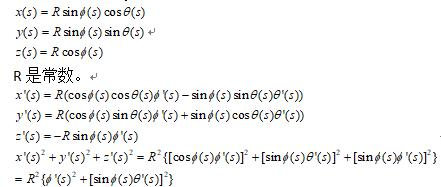所以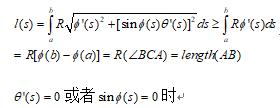上式严格成立，也就是要求不论s取值如何都不能离开大圆弧AB时等式严格成立，这就证明了球面上两点的最短距离为大圆弧。这个距离被高斯称为球面测地线。
展开全文• 两点之间最短距离 这是我的一个测试，也是我学习html的起点，他们说一个合格的程序员必须学会html，我比他们起步晚了一些，可是我认为还来的及，以后我就用html来记录我的学习记录了。 问题的提出...
    两点之间最短距离

这是我的一个测试，也是我学习html的/起点，他们说一个合格的程序员必须学会html，我比他们起步晚了一些，可是我认为还来的及，以后我就用html来记录我的学习记录了。

问题的提出：
在二维平面的n个点上，找出其中的一对点，使得在n个点组成的所有的点中，该点对的距离最小。

一维：最接近点对问题
方法一：（暴力法）
直接使用双层遍历来计算每一对点的距离，另外新建一个变量记录两点之间距离最小的值，但是如此一来时间复杂度就变为O(n2),

方法二：(分治法）
分治法的思路:

划分成子规模点集S1和S2；
找到S1和S2的最小距离d；
合并S1和S2：利用d在S1的(m-d,m]和S2(m,m+d]中找到最大点和最小点，即p3和q3.选出最小距离，完成合并。

m如何选择
m=(max(s)+min(s))/2;

代码：talking is cheap,show me the code;
/*根据算法随便写的，返回的是两个点之间最小的距离，不是两个点的坐标，如果有问题，欢迎指正*/
/*这里的坐标默认为正序的*/
#include<iostream>
#include<vector>
using namespace std;
int search(vector<int> target)
{
int n=target.size();
if(n<2)   //如果分到一个元素就返回最大数值
return INT_MAX;
int mid=n/2;   //中线
vector<int> temp_left;
vector<int> temp_right;   temp_left.insert(temp_left.begin(),target.begin(),target.begin()+mid); //中线分割  temp_right.insert(temp_right.begin(),target.begin()+mid,target.end());
int p=search(temp_left);
int q=search(temp_right);
int d=temp_right.front()-temp_left.back();
cout<<temp_right.front()<<" "<<temp_left.back()<<endl;
return d>min(p,q)?min(p,q):d;}//返回两边和贯穿中间的最小的两点距离
int main(){
int a={-9,-6,0,4,7,9};
vector<int> target(a,a+6);
cout<<search(target);
return 0;
}

二维最接近点的问题：
思路与一维的最接近点对相似。

选取中垂线l：x=m来作为分割直线。
递归的在S1和S2找出其最小距离d1和d2，并设d=min{d1,d2}，S中最接近点对或是d，或者是某个{p，q}
合并起来

// 随便写思路的如果有误，请指正（注意返回的是两点之间的距离，不是坐标）
#include <iostream>
#include <math.h>
#include<algorithm>
using namespace std;
struct point
{
int x, y;
};
double distance(struct point p1, struct point p2)
{
return sqrt(pow(p1.x - p2.x, 2) + pow(p1.y - p2.y,2));
}
double search(struct point p[],int pl,int pr)
{
if (pl == pr)
return INT_MAX;
int mid = (pl + pr) / 2;
double d_left = search(p, pl, mid);
double d_right = search(p, mid + 1, pr);
double temp_d = min(d_left, d_right);
while (abs(p[pl].y - p[mid].y) > temp_d&&pl < pr)//如果仅仅是单边距离就已经大于上一次比较返回的d就没必要在接下来的敌方继续比较了。
pl++;
while (abs(p[pr].y - p[mid].y) > temp_d&&pl < pr)
pr--;
for (int i = pl; i < pr; i++)
{
for (int j = i + 1; j < pr; j++)
{
if (temp_d < abs(p[i].x - p[j].x))//同理与上面的注释
break;
else
temp_d = min(temp_d, distance(p[i], p[j]));//找到最小的距离
}
}
return temp_d;
}
int main()
{
struct point p;
int n;
cin >> n;
for (int i = 0; i < n; i++)
{
cin >> p[i].x;
cin >> p[i].y;
}
cout<<search(p, 0, n - 1);
}




展开全文• 我有个数据框。一个包含properties locations，另一个包含railway stations locations。属性数据框样本(原始数据框由约700行组成)：properties=pd.DataFrame({'propertyID':['13425','32535','43255','52521'],'...
我有两个数据框。一个包含properties locations，另一个包含railway stations locations。属性数据框样本(原始数据框由约700行组成)：properties=pd.DataFrame({'propertyID':['13425','32535','43255','52521'],'lat':[-37.79230,-37.86400,-37.85450,-37.71870],'lon':[145.10290,145.09720,145.02190,144.94330]})火车站数据框样本(原始数据框由约90行组成)：stations=pd.DataFrame({'stationID':['11','33','21','34','22'],'lat':[-37.416861,-37.703293,-37.729261,-37.777764,-37.579206],'lon':[145.005372,144.572524,144.650631,144.772304,144.728165]})我有一个函数来计算两个位置之间的距离from math import radians, cos, sin, asin, sqrtdef haversine(lon1, lat1, lon2, lat2):"""Calculate the great circle distance between two pointson the earth (specified in decimal degrees)"""# convert decimal degrees to radianslon1, lat1, lon2, lat2 = map(radians, [lon1, lat1, lon2, lat2])# haversine formuladlon = lon2 - lon1dlat = lat2 - lat1a = sin(dlat/2)**2 + cos(lat1) * cos(lat2) * sin(dlon/2)**2c = 2 * asin(sqrt(a))r = 6378 # Radius of earth in kilometersreturn c * r我想找到每个属性与所有站点之间的距离。然后选择距离最短的车站。我试图构造一个for循环，但它没有返回最短距离(分钟)lst=[]for stopLat in stations['lat']:for stopLon in stations['lon']:for propLat in properties['lat']:for propLon in properties['lon']:lst.append(haversine(propLon,propLat,stopLon,stopLat))我的最终输出将如下所示。(每个属性都链接到最近的车站)。stationID propertyID11        5252133        1342521        3253534        43255关于如何解决此问题的任何建议都将有所帮助。谢谢解决方案这是一种解决方法，但我首先将两个数据框与一个附加的“键”合并。然后我使用apply计算距离：properties['key'] = 1stations['key'] = 1df = properties.merge(stations,on='key')del df['key']df['distance'] = df.apply(lambda x: haversine(x['lon_x'],x['lat_x'],x['lon_y'],x['lat_y']),axis=1)print(df)df = df.loc[df.groupby("propertyID")["distance"].idxmin()]df = df[['stationID','propertyID']]print(df)第一次打印：propertyID    lat_x     lon_x stationID      lat_y       lon_y   distance0       13425 -37.7923  145.1029        11 -37.416861  145.005372  42.6686391       13425 -37.7923  145.1029        33 -37.703293  144.572524  47.7234062       13425 -37.7923  145.1029        21 -37.729261  144.650631  40.4155073       13425 -37.7923  145.1029        34 -37.777764  144.772304  29.1293384       13425 -37.7923  145.1029        22 -37.579206  144.728165  40.6504365       32535 -37.8640  145.0972        11 -37.416861  145.005372  50.4280786       32535 -37.8640  145.0972        33 -37.703293  144.572524  49.5048077       32535 -37.8640  145.0972        21 -37.729261  144.650631  42.0470568       32535 -37.8640  145.0972        34 -37.777764  144.772304  30.1386849       32535 -37.8640  145.0972        22 -37.579206  144.728165  45.39704710      43255 -37.8545  145.0219        11 -37.416861  145.005372  48.73848711      43255 -37.8545  145.0219        33 -37.703293  144.572524  42.97108312      43255 -37.8545  145.0219        21 -37.729261  144.650631  35.51061613      43255 -37.8545  145.0219        34 -37.777764  144.772304  23.55269014      43255 -37.8545  145.0219        22 -37.579206  144.728165  40.10140715      52521 -37.7187  144.9433        11 -37.416861  145.005372  34.04328016      52521 -37.7187  144.9433        33 -37.703293  144.572524  32.69687517      52521 -37.7187  144.9433        21 -37.729261  144.650631  25.79577418      52521 -37.7187  144.9433        34 -37.777764  144.772304  16.42436419      52521 -37.7187  144.9433        22 -37.579206  144.728165  24.508280第二次印刷：stationID propertyID3         34      134258         34      3253513        34      4325518        34      52521但是根据该输出站34总是最接近的。那是对的吗？编辑：进一步的解释：我曾经试图找到一种方法来将两个数据帧“合并”在一起，而这两个数据帧没有通常用于合并的通用唯一标识符。我还想将一个数据帧的每一行与另一数据帧(在您的情况下，每个工作站具有每个属性)配对，以便能够比较那些条目。在我的研究中，我发现了使用虚拟密钥的巧妙解决方法。在使用的这种解决方法中，我们看到每一行的键都是1，因此每一行将与其他数据帧中的每一行完全匹配我们想要的。使用apply函数，您可以将任何函数逐行应用于数据框。
展开全文• 两点最短距离f1(x){ lx(x) i[lx] j(x[(i+1):(lx-1)]) k return(c(k,x[k])) } f2(y){ n(y) yy(y,1:n) y1(yy[-n,],1,f1) i<-which.R
• Floyd算法求有向图两点间最短距离 问题描述 用Floyd算法求解下图各个顶点的最短距离。写出Floyd算法的伪代码和给出距离矩阵...那么在求有向图中ab两点最短路径时，遍历剩下的点，比较在a到b的路径中是经过Vi距离短...算法
• 基本思想 通过Floyd计算图G=(V,E)中各个顶点的最短路径时，需要引入一个矩阵S，矩阵S中的元素a[i][j]表示顶点i(第i个顶点)到顶点j(第j个顶点)的距离。 假设图G中顶个数为N，则需要对矩阵S进行N次更新。初始时，...学习算法
• 按照象棋中马走的方向,判断两点最短距离或不可到达; http://poj.org/problem?id=2243 #include&lt;iostream&gt; #include&lt;queue&gt; #include&lt;cstring&gt; using namespace std;...
• 实验三：C#实现求图的任意两点最短路径及距离 实验目的 定义Graph类、Node类，从文件中读取图结构；给定两个顶点，给出两个顶点的最短路径（如果没有连通，请给出）。把图进行可视化展示（一个适当大小的图），...C# Floyd算法
• 我们在地图上看航线的时候，特别是长距离跨国航线，发现在地图上的航线并不是两点间的直线，而是弯曲的弧线。...而地球是球形的，很多人没有意识到在球体中，平面上这段距离并不是这两点间的最短距离。我们通过Goog...
• 每周推送到三篇内容上有份量的数学文章，但在行文上力争做到深入浅出。几分钟便可读完，轻松学数学。特别声明，本人未曾授权任何网站(包括微博)、公众号或其他什么号转载北京邵勇原创的“数学教学研究”公众号的...
• 给出一个不带边权（即边权为1）的有向无环图（unweighted DAG）以及DAG上两点s, t，求s到t的最短距离,如果无法从s走到t，则输出-1。 Solution DFS，BFS都可，对于unweighted DAG, BFS更合适，下面给出DFS解法。 ...
•Floyd算法
• 求解城市之间的最短距离是一个非常实际的问题，其大意如下：某地区由n个城市，如何选择路线使某个城市到某个指定城市的的距离最短？注意：这里需要求解的最短路径指的是个城市之间的最短距离，而不是所有城市之间...
• 图论中求任意两点间的最短距离matlab程序实现经典算法
• 就是最长的两点最短距离。也就是说，对于某两个点i和j，它们间的最短距离最大。而这就是无向图的直径。【分析】 一开始我以为是二分，看到“最短距离最大”，我瞬间就想到了“最小值最大”。但是这是错误的思路。...
• 一个最简单的例子：如果你是一个滑雪运动员，目标是最短时间冲线，你根本就不在乎两点间的最短路径，而是最快路径。如果你沿着最佳曲线下滑，你会获得更多的优势……顺势借力　开拓创新从起点到终点，有无数条道路，...
• 题意：给一颗树，求树上两点最短距离。 思路：dist【i】表示根到i的距离。设z为xy的lca，xy的最短距离为dist【x】+dist【y】-2*dist【z】。 #include <iostream> #include <cstdio> #in...
• 我想求得曲面上已知两点间的最小距离。首先根据一系列散点数据，用以下程序拟合出了地形图。A=[55590,86720,1576.3;55730,86770,1588.9;55880,86620,1550.1;56140,86620,1596.8;56220,86370,1592.3;56260,86040,1573...
• 引入向量后，通过将空间元素的位置关系转化为数量关系，将过去的形式逻辑证明转化为数值运算，即借助向量法使解题模式化，用机械性操作把问题转化
• Floyd算法是一种利用动态规划求解的算法，其状态转移方程为...其中map(i , j)表示i到j的最短距离，k为i、j之间的每个。 由下面的例程可知时间复杂度为O(n^3) 例程如下 #define NUM 3 int f_min(int a , int b);算法 graph
• using System; using System.Collections.Generic; using System.Linq; using System.Text;...//迪杰斯特拉算法 计算两点最短距离 namespace Dijkstra { class Program { /// /// 邻接矩阵 ///
• 某地区由n个城市，如何选择路线使某个城市到某个指定城市的的距离最短？ 注意：这里需要求解的最短路径指的是个城市之间的最短距离，而不是所有城市之间最短总距离。 1.最短路径算法 //最短路径算法 ...
• 对于任何一个而言，i到j的最短距离不外乎存在经过i与j之间经过k和不经过k种可能，所以可以令k=1，2，3，…，n(n是节点的数目)，在检查d(ij)与d(ik)+d(kj)的值；在此d(ik)与d(kj)分别是目前为止所知道的i到k与k到......